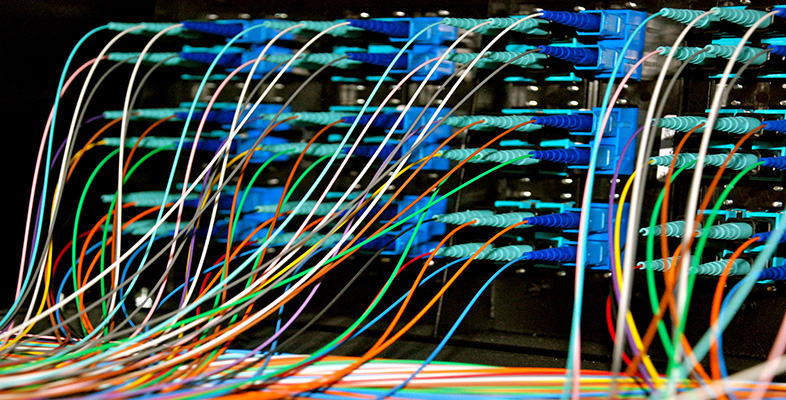Representing and manipulating data in computers

This free course is available to start right now. Review the full course description and key learning outcomes and create an account and enrol if you want a free statement of participation.

Free course

# 2.2 Representing numbers: positive integers

A very straightforward way of finding binary codes to represent positive integers is simply to use the binary number that corresponds to each integer. This is because every positive integer in the everyday number system (known as the decimal or denary system because it uses 10 different digits) has a corresponding number in the binary number system.

As you will see later, in Section 7 of this course, just as arithmetic (addition, subtraction, etc.) can be performed on everyday denary numbers, so it can also be performed on binary numbers. This means that encoding denary numbers as binary numbers is particularly useful whenever an application will include arithmetic. And this is the case with the kitchen scales, thanks to their add-and-weigh function.

You need to know something about binary numbers, and so in this section I shall first remind you of an important principle of the number system you are already familiar with, the 10-digit decimal or denary system, then show how that principle applies to the 2-digit binary number system. I'll also show you how to convert between the two systems.

T224_2